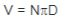# Formulas

### The following formulas cover the basic calculations used in brake application engineering.

REQUIRED GIVEN FORMULA
Full load motor torque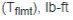Horsepower (P), hp
Shaft speed (N), rpm
5252 = Constant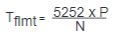Average dynamic braking torque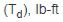Total inertia reflected to brake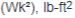Shaft speed at brake (N), rpm
Desired stopping time (t), seconds
308 = Constant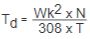Static torque (T), lb-ft
Force (F), lb
Pulley or drum radius, (R), ft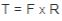Overhauling dynamic torque reflected to brake shaft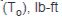Weight of overhauling load (W), lb
Linear velocity of descending load (V), ft/min
Shaft speed at brake (N), rpm
0.158 = Constant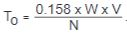Static torque of brake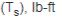Dynamic braking torque required0.8 = Constant (derating factor)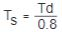Inertia of rotating load reflected to
brake shaft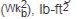Inertia of rotating load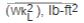Shaft speed at loadShaft speed at brake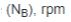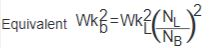Equivalent inertia of linear moving load
reflected to brake shaft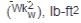Weight of linear moving load (W), lb
Linear velocity of load (V), ft/min
Shaft speed at brake2 = Constant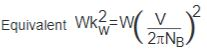Kinetic energy of rotating load,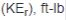Inertia of rotating load reflected to brake shaftShaft speed at brake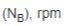5875 = Constant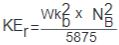Kinetic energy of linear moving load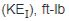Linear velocity of load (v), ft/sec
g = Gravitational acceleration constant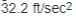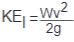Change in potential energy (PE), ft-lb
Weight of overhauling load (W), lb
PE = Ws
Total energy absorbed by brake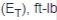Total linear kinetic energy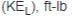Total rotary kinetic energy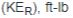Potential energy converted to kinetic energy
(PE), ft-lb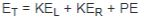Thermal capacity required for rotational or linear
Total system inertia reflected to brake shaft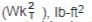Shaft speed at brakeNumber of stops per minute (n), not less
than one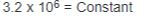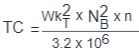Thermal capacity required for overhauling loads
(TC), hp-sec/min
Total energy brake absorbsNumber of stops per minute (n), not less
than one 550 = ConstantLinear velocity, ft/min
N = rpm
Diameter (D), ft